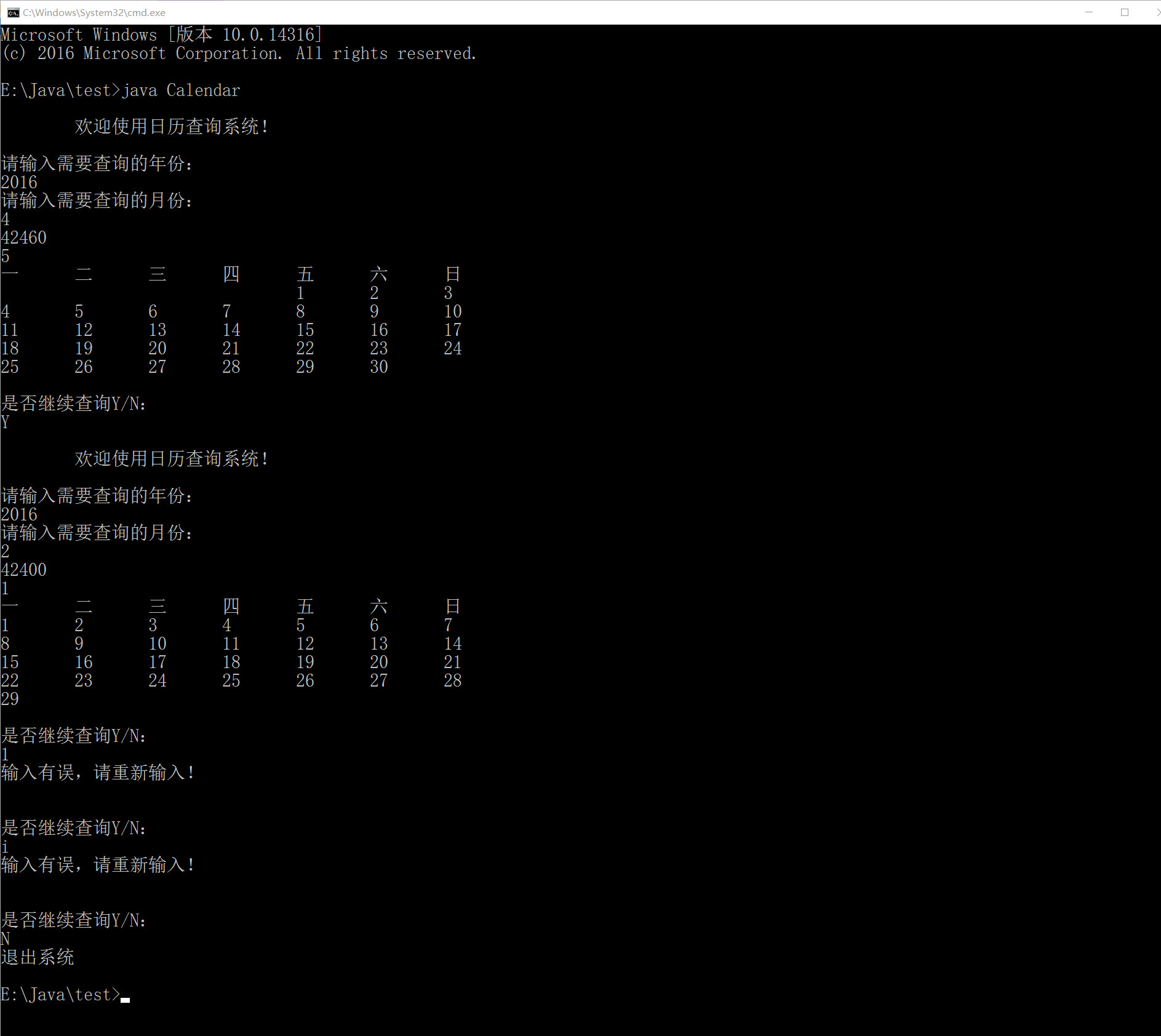# Java 日历的制作 心得 写给自己

1. 写方法的时候忘记加（），尤其是在写构造方法的时候，需要留意代码的完整性​
2. {}没有配套，虽然这个只是个书写问题，但是也是需要留意的问题​
3.  使用Scanner new 对象的时候忘记在（）里面加System.in
4. 写使用对象引用方法的时候常常忘记加（）


 1. 谨记在条件语句中使用键盘输入的禁忌，一定不能直接在条件语句中使用键盘输入，一定要将键盘输入赋值给一个变量，将这个变更代入到条件语句中，否则将不停地输入，使程序无法继续进行
2. 当需要进行循环时不知道什么需要需要跳出（可能是视用户情况来决定，例如用户输入），可以尝试先boolean一个变量为true，当循环进入尾声时，需要用户输入选择是否继续循环，此时可以按需要当boolean变量的值改为true或false，这样就可以跳出循环
3. 使用try catch来提示用户输入错误类型的值，例如String A = in.next()；但是如果用户输入了一个int型的值，那么可以用try catch来提示用户输入有误
4. 结束循环可以用continue或break，但是他们是不同的，continue是跳出本次循环，break是直接结束循环语句
5. 当一个对象不再使用的时候可以当其设置为null，这个JAVA会自动将内存回收
6. 注意&&与||的顺序，如果顺序不同，那么他们的逻辑也不同，结果也将会不同
7. 设置方法的时候如何确定是否需要返回？如果需要在其他类与其他方法里面获得一个值，那么就需要用返回。那么返回的是什么呢，return的是什么就返回什么。（对于return的理解感觉还不够到位，后续还需要请教或继续观察这个问题）
8. 封装的操作有待加强


//Cal类
class Cal
{

Cal()
{
System.out.println("\n\t欢迎使用日历查询系统！\n");
}

private int year;
private int month;
private int sum;

public void setYear(int year)
{
this.year = year;
}
public int getYear()
{
return year;
}

public void setMonth(int month)
{
this.month = month;
}
public int getMonth()
{
return month;
}

public void setSum(int sum)
{
this.sum = sum;
}

public int getSum()
{
return sum;
}

public void getDays(int year,int month)
{
this.setSum(0);

for (int i = 1900;i<year ;i++ )
{
if (i%4==0&&i%100!=0||i%400==0) //注意&&与||的顺序，如果顺序不同，那么他们的逻辑也不同，结果也将会不同
{
sum+=366;
}
else
{
sum+=365;
}
}

for (int i = 1;i<month ;i++ )
{
if (i==2)
{
if (year%4 == 0&&year%100 != 0||year%400==0 )
{
sum+=29;
}
else
{
sum+=28;
}
}
else
{
if (i==4||i==6||i==9||i==11)
{
sum+=30;
}
else
{
sum+=31;
}
}
}

sum+=1; // 自加1是为了自动跳到下一个月的第一天（因为上面的for循环中是<month，不是<=month）
}

public int getWeekNum()
{
int W = this.getSum()%7;
return W;
}

public void prepare()
{
System.out.println("一\t二\t三\t四\t五\t六\t日");

if (this.getWeekNum()==0)
{
System.out.print("\t\t\t\t\t\t");
}
else
{
for (int i=1;i<this.getWeekNum() ;i++ )
{
System.out.print("\t");
}
}
}

public void outPut(int year,int month)
{

if (month==2)
{
if (year%4==0&&year%100!=0||year%400==0)
{
for (int i = 1;i<=29 ;i++ )
{
if (this.getWeekNum()!=0)
{
System.out.print(i+"\t");
}
else
{
System.out.print(i+"\n");
}
sum++;
}
}
else
{
for (int i = 1;i<=28 ;i++ )
{
if (this.getWeekNum()!=0)
{
System.out.print(i+"\t");
}
else
{
System.out.print(i+"\n");
}
sum++;
}

}
}
else
{
if (month == 4||month == 6||month == 9||month == 11)
{
for (int i = 1;i<=30 ;i++ )
{
if (this.getWeekNum()!=0)
{
System.out.print(i+"\t");
}
else
{
System.out.print(i+"\n");
}
sum++;
}
}
else
{
for (int i = 1;i<=31 ;i++ )
{
if (this.getWeekNum()!=0)
{
System.out.print(i+"\t");
}
else
{
System.out.print(i+"\n");
}
sum++;
}
}
}
}
}
//主程序
import java.util.Scanner;
public class Calendar
{
public static void main(String[] args)
{
Scanner in = new Scanner(System.in);
boolean circle = true;
while(circle)
{
Cal C = new Cal();
System.out.println("请输入需要查询的年份：");
C.setYear(in.nextInt());
System.out.println("请输入需要查询的月份：");
C.setMonth(in.nextInt());

C.getDays(C.getYear(),C.getMonth()); //计算SUM求得总天数；
System.out.println(C.getSum()); // 输出总日期时间；
System.out.println(C.getWeekNum()); // 计算W的值，确定输入月份的1号是星期几；
C.prepare(); //输出日历框架；
//System.out.println(C.prepare()); // 计算W的值，确定输入月份的1号是星期几；
C.outPut(C.getYear(),C.getMonth()); // 输出日历详细
C = null;

while(circle) //小循环系统，确认是否继续查询
{
System.out.println("\n\n是否继续查询Y/N：");
try // try catch 语句，用于提示错误的输入类型
{

/*
* 非常重要！！！！！！！！！！！！！！！！！！！！
* 谨记在条件语句中使用键盘输入的禁忌，一定不能直接在条件语句中使用键盘输入，
* 一定要将键盘输入赋值给一个变量，将这个变更代入到条件语句中，否则将不停地输入
*/

String continu = in.next();

if (continu.equals("Y")||continu.equals("y"))
{
break; //结束这个循环（continue是跳出这一次循环，注意区别）
}
else
{
if (continu.equals("N")||continu.equals("n"))
{
System.out.println("退出系统");
circle = false; // 将circle赋为false 就等于是从源头上结束循环语句
}
else
{
System.out.println("输入有误，请重新输入！");
}
}

}
catch (Exception e)
{
System.out.println("输入有误"); //如果有输入错误类型的情况，将提示输入错误
}
}
}
}
}